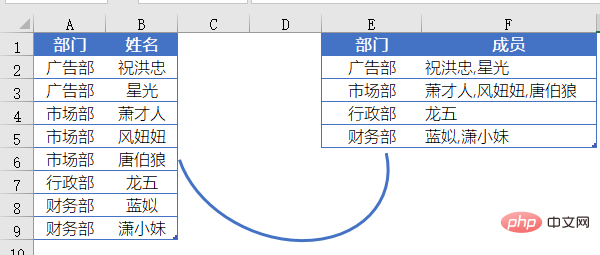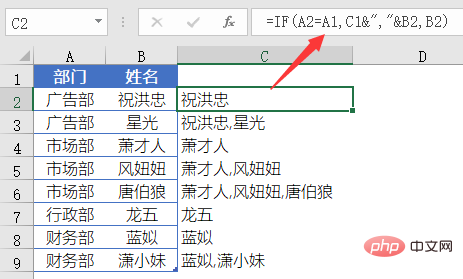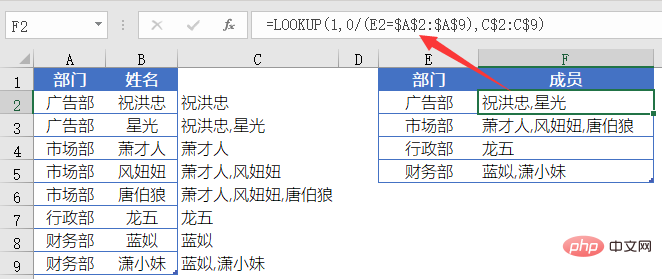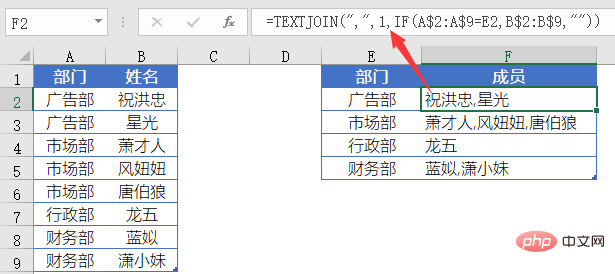# Excel怎么把数据合并到一个单元格A列是某公司部门名称，B列是人员姓名。

`=IF(A2=A1,C1&","&B2,B2)`F2输入公式：

`=LOOKUP(1,0/(E2=\$A\$2:\$A\$9),C\$2:C\$9)`C列为辅助列，是一个简单的IF函数。

`=IF(A2=A1,C1&","&B2,B2)`

`=IF(A3=A2,C2&","&B3,B3)`

A3和A2的值相等，返回真值C2&”,”&B3。

C2为上个公式所返回的结果B2（祝洪忠），B3的值是”星光”，所以C3最后结果为”祝洪忠,星光”。

`=LOOKUP(1,0/(E2=\$A\$2:\$A\$9),C\$2:C\$9)`

LOOKUP的这个套路，忽略错误值，总是取得最后一个符合条件的结果，我们可以总结为：

`=LOOKUP(1,0/(条件区域=指定条件),要返回的目标区域)`

`=TEXTJOIN(",",1,IF(A\$2:A\$9=E2,B\$2:B\$9,""))`TEXTJOIN函数的用法为：

`=TEXTJOIN(间隔符号,是否忽略空单元格,要合并的内容)`

IF(A\$2:A\$9=E2,B\$2:B\$9,"")

{“祝洪忠”;”星光”;””;””;””;””;””;””}

TEXTJOIN函数对IF函数得到的内存数组进行合并，第一参数指定使用间隔符号为逗号，第二参数使用1，表示忽略内存数组中的空文本。• 相关标签：Excel
• 程序员必备接口测试调试工具：点击使用

Apipost = Postman + Swagger + Mock + Jmeter

Api设计、调试、文档、自动化测试工具

后端、前端、测试，同时在线协作，内容实时同步

支持grpc,http,websocket,socketio,socketjs类型接口调试相关文章

相关视频

## 专题推荐

官方公众号

php中文网课程

扫码关注官方公众号
回复“phpcn01”领取php学习资料课程
全套工具、电子书、脑图、内部课程等